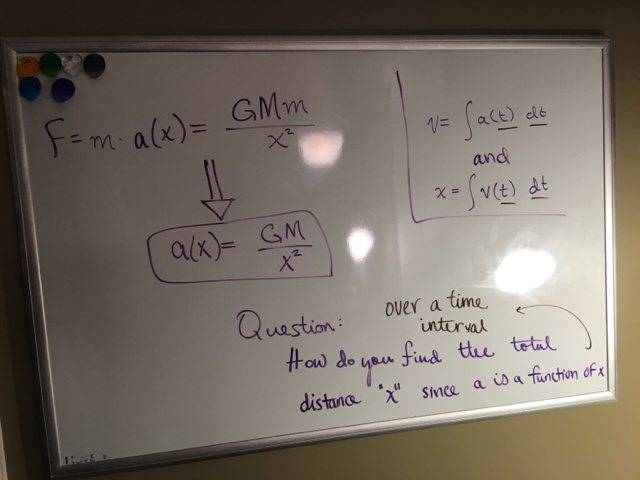# Acceleration as a function of x to a function of timeBvU
Homework Helper
In 1 dimension ?

In 1 dimension ?

Yes

BvU
Homework Helper
And is $$F = -\displaystyle {GMm\over x^2}$$ or is $$F = +\displaystyle{GMm\over x^2}$$ as on the whyteboard ?

And is $$F = -\displaystyle {GMm\over x^2}$$ or is $$F = +\displaystyle{GMm\over x^2}$$ as on the whyteboard ?

Positive

BvU
Homework Helper
I didn't do anything except enter the thing in wolframalpha !

I didn't do anything except enter the thing in wolframalpha !

So what does that equation mean?

Chestermiller
Mentor
$$\frac{dv}{dt}=-\frac{GM}{x^2}$$If you multiply both sides of this equation by v=dx/dt, you get:$$v\frac{dv}{dt}=-\frac{MG}{x^2}\frac{dx}{dt}$$Both sides of this equation are exact differentials with respect to time.

$$\frac{dv}{dt}=-\frac{GM}{x^2}$$If you multiply both sides of this equation by v=dx/dt, you get:$$v\frac{dv}{dt}=-\frac{MG}{x^2}\frac{dx}{dt}$$Both sides of this equation are exact differentials with respect to time.

So is the following correct?

$$v dv = \frac{-MG}{x^2} dx$$

Chestermiller
Mentor
So is the following correct?

$$v dv = \frac{-MG}{x^2} dx$$
Yes.

Yes.

So how do you integrate over a time interval? That is to say, how do you find the velocity over the interval [0, t]?

Chestermiller
Mentor
So how do you integrate over a time interval? That is to say, how do you find the velocity over the interval [0, t]?
Do you know how to solve for v as a function of x?